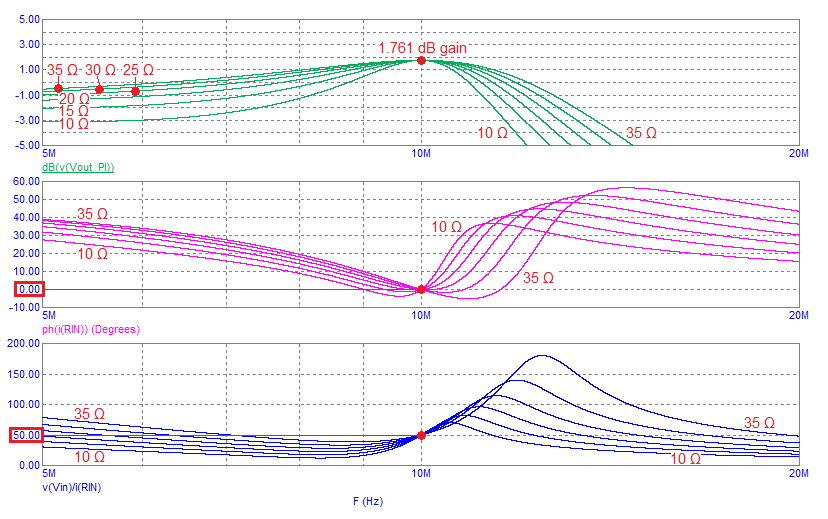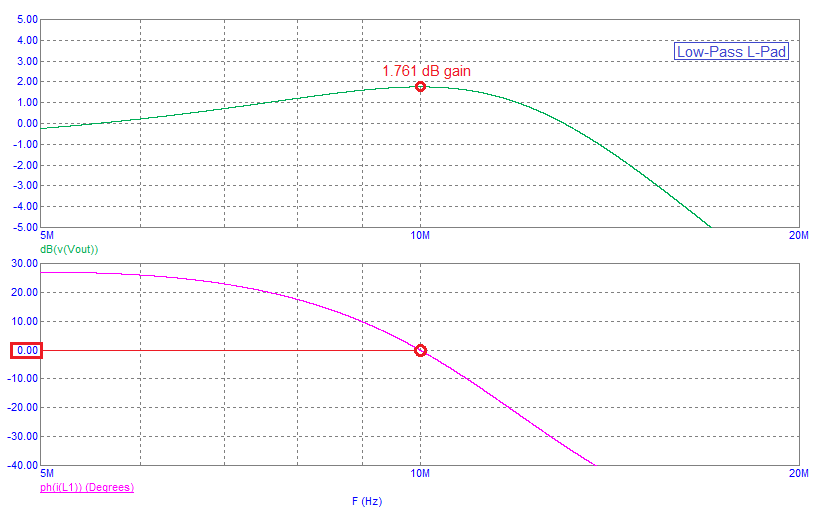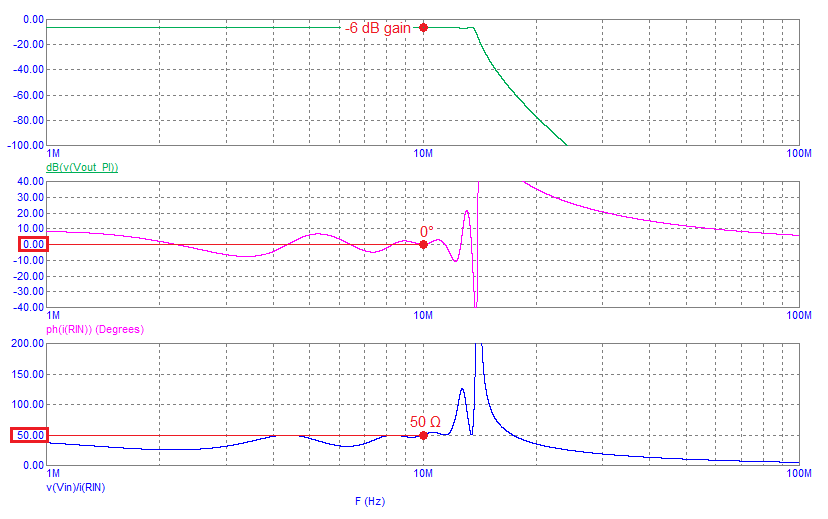LOW PASS $$\pi$$ MATCHINGThe low-pass $$\pi$$ network is equivalent to a pair of back-to-back low-pass L-Pad networks: -

$$\hspace{18.9cm}$$

Low-pass $$\pi$$ network

Equivalent circuit$$C_1 = \dfrac{1}{\omega\cdot R_{IN}}\sqrt{\dfrac{R_{IN}}{\color{red}{R_X}}-1}\hspace{1cm} L_1 = C_1\cdot R_{IN}\cdot\color{red}{R_X}$$

$$C_2 = \dfrac{1}{\omega\cdot R_{L}}\sqrt{\dfrac{R_{L}}{\color{red}{R_X}}-1}\hspace{1.7cm} L_2 = C_2\cdot R_{L}\cdot\color{red}{R_X}$$

The equivalent circuit

RX represents $$R_L$$ for the left-stage and $$R_{IN}$$ for the right stage. You solve the left and right stages independently then combine L1 and L2 into inductor LT. RX is called the transfer impedance $$Z_T$$.

The calculator below requires RX to be less than RIN. This makes L1 and C1 an impedance reducer with L2 and C2 as an impedance multiplier. With the $$\pi$$ calculator's default values, 50 Ω is converted to a $$Z_T$$ of 25 Ω and then $$Z_T$$ is multiplied to 300 Ω.

 RIN: Ω RX: Ω RL: Ω FIN: MHz L1: µH L2: µH LT: µH C1: pF C2: pF AV at FIN: Voltage gain from $$Z_{IN}$$ to $$R_L$$ V/V

The relevance of Q-factor

As previously proven for the basic L-Pad network, these relations are all true: - $$Q \hspace{0.5cm} = \hspace{0.5cm}A_V\hspace{0.5cm} = \hspace{0.5cm}\sqrt{\dfrac{R_L}{R_{IN}}}\hspace{0.5cm} = \hspace{0.5cm}R_L\sqrt{\dfrac{C}{L}}\hspace{0.5cm}=\hspace{0.5cm}\omega_n R_L C$$

This means that Q is not an input variable; it is wholly defined by $$R_{IN}$$ and $$R_L$$. However, because the $$\pi$$ network has a transfer impedance, Q-factor has two identities. For this reason, Q is discarded as an input variable in favour of RX. $$A_V$$ is still calculated based on a lossless power transfer: - $$A_V=\sqrt{\dfrac{R_L}{R_{IN}}}$$

Benefits of the $$\pi$$ network

The 1st benefit is that you can manipulate the pass-bandwidth at the operating frequency. The image below shows the bode-plot response for several values of RX. The upper graph is gain and the lower graphs are $$\angle{Z_{IN}}$$ and $$|Z_{IN}|$$: -At 10 MHz, the gains converge at 1.761 dB. In real numbers that's 1.2247 and, when taking into account the 2:1 loss when connecting a 50 Ω source to a network with $$Z_{IN}$$ = 50 Ω, the voltage magnification is the same as the low-pass L-Pad network (2.44949): -The 2nd benefit; The input impedance phase angle can be tailored to be more closely resistive than the L-Pad in the operating region around the centre frequency.

The 3rd benefit is that the input impedance and output impedance can be equal. This may not seem much of a benefit for an impedance transformer but, if you consider that several $$\pi$$ networks can be cascaded to produce a significant filtering effect, you have a tool that is very useful.

The low-pass $$\pi$$ network in cascade

Below is a 50 Ω to 50 Ω, 5-stage cascaded $$\pi$$ network simulated in Micro-cap 12 (RX = 25 Ω): -The frequency response, impedance phase angle and impedance magnitude is this: -The main feature is the very sharp attenuation from about 13 MHz. The impedance is fairly flat from about 8 MHz to 11 MHz with the corresponding impedance phase angle remaining close to 0°.

When cascading $$\pi$$ stages, the value calculations are repeated and trivial.